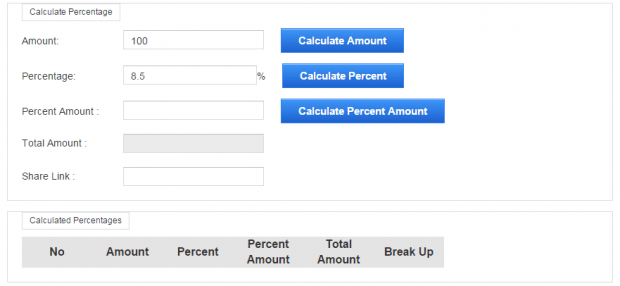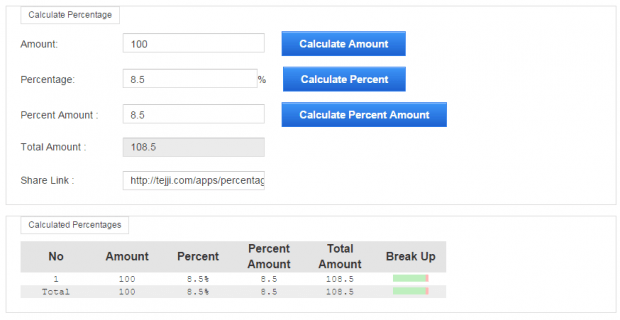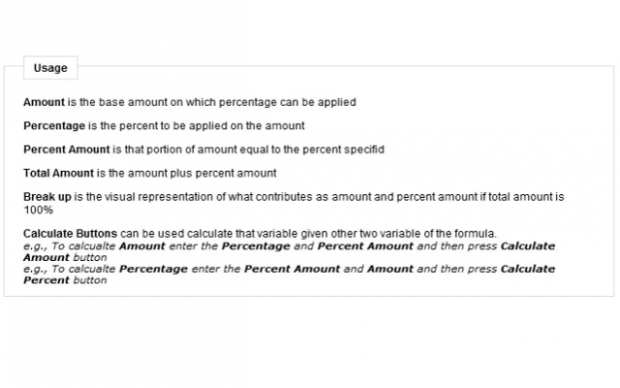# How to calculate percentage value in Chrome [Tip]

If Google Search is your default search engine, then you can easily make calculations just by entering your desired formula in the search bar. For example, you can do addition or subtraction. You can even convert values and calculate percentages.

As for the latter, just use the P% * X = Y formula to find the percentage of a number. Type it on the search bar and then press “Enter”. It’s really easy but what if you need to get the percentage of multiple values? If that is the case, then it would be best to have a handy percentage value calculator right within your web browser.

If you’re a Chrome user, then you can follow the steps listed below.

### How to calculate percentage value in Chrome

• First, you’ll need to download the “Percentage Calculator” browser app from the Chrome Web Store. Install it on your browser.• After installing Percentage Calculator, open it from your Chrome app launcher.• On the calculator, type the base amount and percentage. For example, if you want to know how much is 8.5% in 100, just type “100” on the “Amount” field and then “8.5” on the “Percentage” field.• Click “Calculate Percent Amount” to get the answer.
• With Percentage Calculator, you can get both the percentage amount and the total amount. You can also track your recent calculations in the table provided.So that’s it.

With the said app, you can easily calculate multiple percentage values. It’s a handy tool especially if you are constantly working on commission reports, product discounts, etc.